# Formal group

(diff) ← Older revision | Latest revision (diff) | Newer revision → (diff)

An algebraic analogue of the concept of a local Lie group (cf. Lie group, local). The theory of formal groups has numerous applications in algebraic geometry, class field theory and cobordism theory.

A formal group over a fieldis a group object in the category of connected affine formal schemes over(see , , , ). Let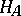be the functor that associates with an algebrathe set of algebra homomorphism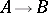from some Noetherian commutative local-algebrawith maximal idealand field of residues, complete in the-adic topology, such that the homomorphisms mapinto the set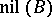of nilpotent elements of. Then a connected affine formal scheme is a covariant functorfrom the category of finite-dimensional commutative-algebrasinto the category of sets that is isomorphic to an. Thatis a group object means that there is a group structure given on all the sets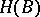such that for every-algebra homomorphism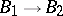the corresponding mapping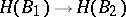is a group homomorphism. If all the groupsare commutative, then the formal groupis said to be commutative. Every connected group schemeoverdefines a formal group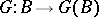. Here one can take asthe completion of the local ring ofat the unit element.

Ifis the ring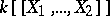of formal power series invariables over, thenis called an-dimensional formal Lie group. For a connected algebraic groupover,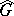is a formal Lie group. A formal Lie groupis isomorphic, as a functor in the category of sets, to the functor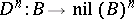that associates with an algebrathe-fold Cartesian product of its nil radicalwith itself. The group structure on the sets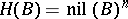is given by a formal group law — a collection offormal power series invariables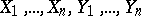: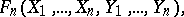satisfying the following conditions: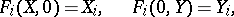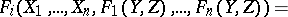Here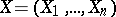,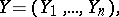,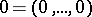. This group law on the setsis given by the formulas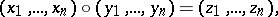where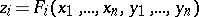; becauseandare nilpotent, all except a finite number of terms of the series are zero. Every formal group law gives group structures on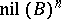by means of

and converts the functorinto a formal Lie group. The concept of a formal group law, and thus of a formal Lie group, can be generalized to the case of arbitrary commutative ground rings (see , ). Sometimes by a formal group one means just a formal Lie group or even a formal group law.

Just as for local Lie groups (cf. Lie group, local) one can define the Lie algebra of a formal Lie group. Over fieldsof characteristic 0 the correspondence between a formal Lie group and its Lie algebra defines an equivalence of the respective categories. In characteristic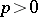the situation is more complicated. Thus, over an algebraically closed field (for) there is a countable number of pairwise non-isomorphic one-dimensional commutative formal Lie groups , while all one-dimensional Lie algebras are isomorphic . Over perfect fields of finite characteristic, commutative formal Lie groups are classified by means of Dieudonné modules (see , ).

The theory of formal groups over fields can be generalized to the case of arbitrary formal ground schemes .

How to Cite This Entry:
Formal group. Encyclopedia of Mathematics. URL: http://encyclopediaofmath.org/index.php?title=Formal_group&oldid=17665
This article was adapted from an original article by Yu.G. Zarkhin (originator), which appeared in Encyclopedia of Mathematics - ISBN 1402006098. See original article